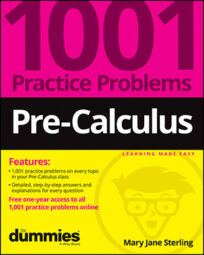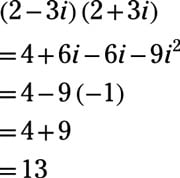##### Pre-Calculus: 1001 Practice Problems For Dummies (+ Free Online Practice)Complex numbers are unreal. Yes, that’s the truth. A complex number has a term with a multiple of i, and i is the imaginary number equal to the square root of –1. Many of the algebraic rules that apply to real numbers also apply to complex numbers, but you have to be careful because many rules are different for these numbers.

You’ll work on complex numbers in the following ways:

• Simplifying powers of i into one of four values

• Adding and subtracting complex numbers by combining like parts

• Multiplying complex numbers and simplifying resulting powers of i

• Dividing complex numbers by multiplying by a conjugate

When working with complex numbers, some challenges will include the following:

• Multiplying imaginary numbers correctly

• Choosing the correct conjugate and simplifying the difference of squares correctly when dividing complex numbers

## Practice problems

1. Write the power of i in its simplest form: i301

Rewrite the exponent as the sum of a multiple of 4 and a number between 0 and 3: i301 = i75(4) + 1

Now write the power of i as the product of two powers: i75(4) x i1

The value of i4n is 1, so i301 = 1 x i1 = i.

2. Multiply. Write your answer in a + bi form: (2 – 3i)(2 + 3i)

Use FOIL to multiply the binomials: